UIHistories Project: A History of the University of Illinois by Kalev Leetaru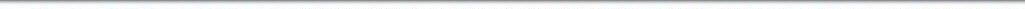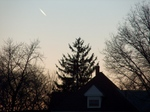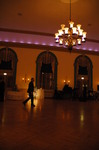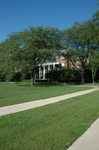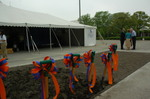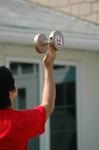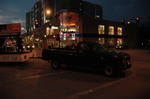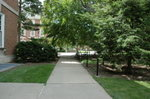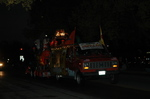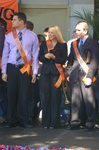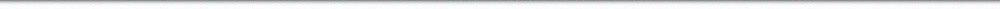N A V I G A T I O N D I G I T A L L I B R A R Y

## Repository: UIHistories Project: Board of Trustees Minutes - 1896 [PAGE 181]

Caption: Board of Trustees Minutes - 1896
This is a reduced-resolution page image for fast online browsing.

< Previous Page [Displaying Page 181 of 371] Next Page >
[VIEW ALL PAGE THUMBNAILS]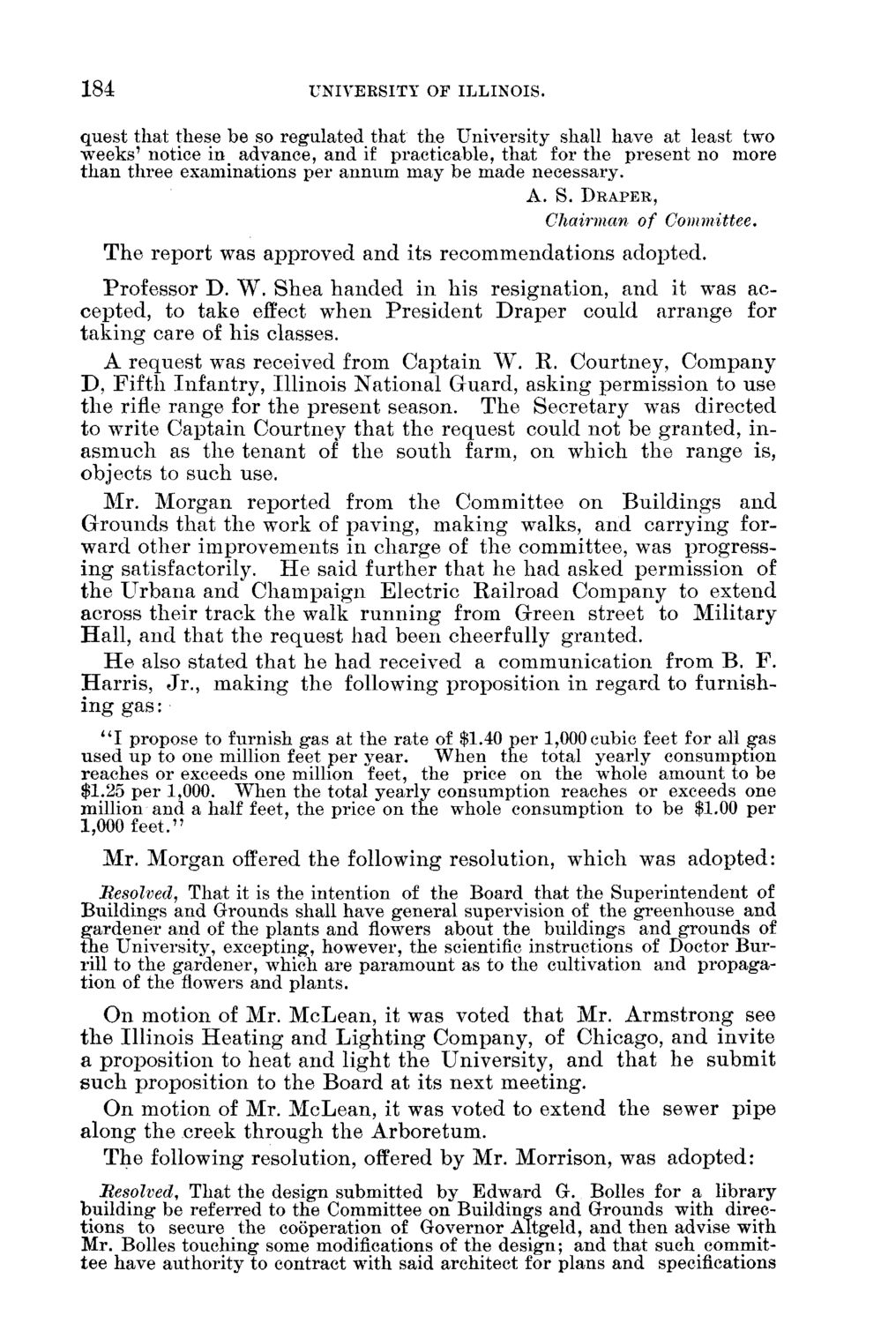### EXTRACTED TEXT FROM PAGE:

184

UNIVEESITY OF ILLINOIS.

quest that these be so regulated that the University shall have at least two weeks' notice in advance, and if practicable, that for the present no more than three examinations per annum may be made necessary.

A. S. DRAPER,

Chairman of Committee. T h e r e p o r t was approved a n d its r e c o m m e n d a t i o n s adopted. Professor D . W . S h e a h a n d e d in h i s resignation, a n d it was accepted, to take effect w h e n P r e s i d e n t D r a p e r could a r r a n g e for t a k i n g care of h i s classes. A r e q u e s t was received from C a p t a i n W . R. C o u r t n e y , C o m p a n y D , F i f t h I n f a n t r y , I l l i n o i s N a t i o n a l G u a r d , a s k i n g p e r m i s s i o n to use t h e rifle r a n g e for t h e p r e s e n t season. T h e S e c r e t a r y was d i r e c t e d to wTrite C a p t a i n C o u r t n e y t h a t t h e r e q u e s t could n o t be g r a n t e d , ina s m u c h as t h e t e n a n t of t h e s o u t h farm, on w h i c h t h e r a n g e is, objects to such use. M r . M o r g a n r e p o r t e d from t h e C o m m i t t e e on B u i l d i n g s a n d G r o u n d s t h a t t h e work of p a v i n g , m a k i n g walks, a n d c a r r y i n g forw a r d o t h e r i m p r o v e m e n t s in c h a r g e of t h e committee, wTas p r o g r e s s i n g satisfactorily. H e said f u r t h e r t h a t he h a d asked p e r m i s s i o n of t h e U r b a n a a n d C h a m p a i g n E l e c t r i c R a i l r o a d C o m p a n y to e x t e n d across t h e i r t r a c k t h e walk r u n n i n g from G r e e n street to M i l i t a r y H a l l , a n d t h a t t h e r e q u e s t h a d been cheerfully g r a n t e d . H e also s t a t e d t h a t h e h a d received a c o m m u n i c a t i o n from B . F . H a r r i s , J r . , m a k i n g t h e following p r o p o s i t i o n in r e g a r d to f u r n i s h ing gas: " I propose to furnish gas at the rate of \$1.40 per 1,000 cubic feet for all gas used up to one million feet per year. When the total yearly consumption reaches or exceeds one million feet, the price on the whole amount to be \$1.25 per 1,000. When the total yearly consumption reaches or exceeds one million and a half feet, the price on the whole consumption to be \$1.00 per 1,000 feet." M r . M o r g a n offered t h e following resolution, w h i c h was a d o p t e d : Resolved, That it is the intention of the Board that the Superintendent of Building's and Grounds shall have general supervision of the greenhouse and gardener and of the plants and flowers about the buildings and grounds of the University, excepting, however, the scientific instructions of Doctor Burrill to the gardener, which are paramount as to the cultivation and propagation of the flowers and plants. O n motion of Mr. M c L e a n , it was voted t h a t Mr. A r m s t r o n g see t h e Illinois H e a t i n g a n d L i g h t i n g C o m p a n y , of Chicago, a n d invite a p r o p o s i t i o n to h e a t a n d l i g h t t h e U n i v e r s i t y , a n d t h a t h e s u b m i t s u c h proposition to t h e B o a r d at its n e x t m e e t i n g . O n m o t i o n of Mr. M c L e a n , it was voted to extend t h e sewer p i p e a l o n g t h e creek t h r o u g h t h e A r b o r e t u m . T h e following resolution, offered by Mr. Morrison, was a d o p t e d : 'Resolved, That the design submitted by Edward G. Bolles for a library building be referred to the Committee on Buildings and Grounds with directions to secure the cooperation of Governor Altgeld, and then advise with Mr. Bolles touching some modifications of the design; and that such committee have authority to contract with said architect for plans and specifications